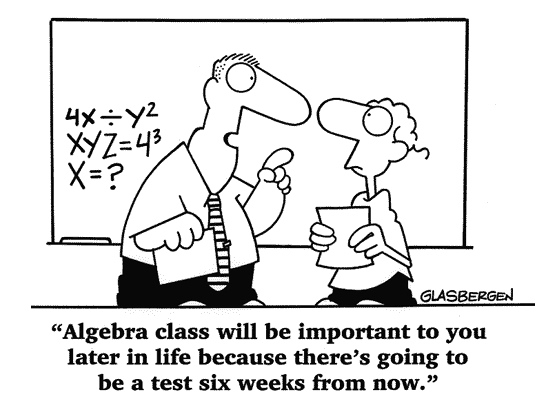Learn the 4 Things You Must Know Before Taking the GMAT!   Download it Now!I want to get back to the basics in this blog post. As you’ve probably noticed in your GMAT prep, a lot of times you have to do basic math in the context of solving more difficult or complex GMAT math problems. We spend a lot of time teaching you how to solve those more difficult problems (tricks, strategies, methodology, etc.), but I’ve noticed that some of my students forget their math fundamentals. Let’s look, for example, at how to solve for x in an algebraic expression that contains fractions.

Example: Solve for x in the following equation

1/3 x + 2 = 2/5 x + 4

There are four simple steps to follow in solving any algebraic equation. Pay particular attention to Step 1 on equations that involve fractions or decimals.

Step 1: If there are fractions or decimals, remove them by multiplication

In this example, the best way to get rid of both fractions at once is to multiply both sides of the equation by the least common denominator (for review, see the video lesson on “Percents, Fractions, Decimals…“). The least common denominator of 1/3 and 2/5 is 15. So,

 1/3 x + 2 = 2/5 x + 4   ==>
5x + 30 = 6x + 60

Step 2: Collect all terms containing the variable for which you are solving on the same side of the equation (I recommend collecting them on the left side of the equal sign)

To do so, simply subtract 6x from the right side of the equation and subtract 30 from the right side of the equation. The result is

5x – 6x = 60 – 30

Step 3: Determine the coefficient of the variable (i.e. the number that comes immediately before the variable you’re solving for) by combining similar terms or factoring when terms cannot be combined.

-1x = 30

The coefficient, therefore, is -1.

Step 4: Divide both sides of the equation by the coefficient

x = -30

That’s about it. Pretty basic, but sometimes it’s nice to dust off those “math cobwebs.” The GMAT can be difficult enough without worrying about whether you’re even working with the right “x,” so to speak. I hope this helps, and go out and dominate the GMAT!